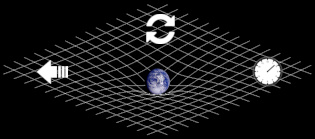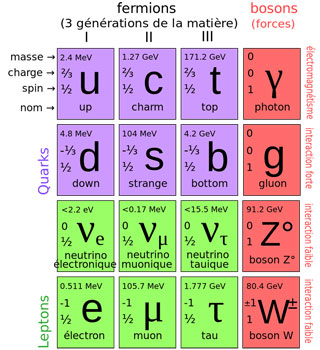# Symmetries of the universe

## Concept of symmetry in physicsAutomatic translation Updated November 13, 2021

Everyone knows what the symmetry of an object is, it is its reflection in a mirror. That is to say the image of the object where the notions of right and left are reversed.
But what is a symmetry in the universe?
It is a transformation that leaves an object unchanged. A physical system is symmetric if it remains invariant under the action of any transformation operation.
To understand, imagine an empty universe and throw an object into the void of space. The object will acquire a movement and at each moment its position will change. As the laws of physics are invariant in space and time, then the situation of the object at time t is equivalent to the situation of the object at time t + 1, t + 2, t + 3, etc. Since all situations are the same, the momentum is going to be preserved forever.
In physics, in the absence of external influence, all bodies continue in a uniform rectilinear motion. This translational symmetry forces the object to keep its movement, which explains why the object moves in a straight line at constant speed. This thought experiment can be done with a rotating object. Whether we turn the object or give it another orientation the result remains the same, we will then have rotational symmetry.
If we carry out the experiment at another time the result remains the same, we will then have symmetry in time.

The symmetries of the universe force objects to keep their movement. But each symmetry imposes the conservation of a quantity over time.
For translational symmetry, this quantity is the momentum. For rotational symmetry, this quantity is the angular momentum. For temporal symmetry, this quantity is energy (Noether's theorem).
Noether's theorem also applies to the quantum field like the field of electrons.
Likewise, the laws of physics that describe electrons do not change when the phase of all complex numbers in the field is rotated at the same time. The retained magnitude is the electric charge.
According to Noether's theorem, it is only when the universe has symmetry that these quantities are preserved.

NB: Noether's theorem - To any infinitesimal transformation which leaves the action integral invariant corresponds a quantity which is conserved.
Amalie Emmy Noether (1882 - 1935) German mathematician specializing in abstract algebra and theoretical physics.## What are symmetries for?

Our real universe does not seem symmetrical, it is not the same everywhere, it contains stars and planets. It does not appear to be symmetrical over time since it swells. If we throw an object on Earth its momentum is not conserved, it speeds up or slows down. Yet the laws of physics do not change!
To keep the absolute character of the laws of physics, it was necessary to add a structure, an entity or a force field to the laws.
This is how the concept of curvatures of space-time appeared in the theory of general relativity (Rμν-1 / 2gμνR = (8πG / c4) Tμν). This structure makes it possible to describe the universe from any point of view.
In particle physics, it was necessary to add fields (fields of fundamental interactions) to restore the invariance of the laws.
It is the same for the laws which describe the electrons (L = ψ (iδ-m) Ψ + JμδμΦ). If we want to restore symmetry, whatever the reference level we choose, we must introduce a kind of force field with which the electron field interacts. This field is the electromagnetic field which contains particles (photons) interacting with electrons.
It is because the universe has symmetries that the objects it contains obey laws. Symmetries allow us to understand the construction of physical theories.

By considering the laws of physics as absolute, we could imagine the presence of new structures (curvature of space-time, Higgs field, electromagnetic field, etc.).
By measuring certain properties of the particles, the symmetries made it possible to understand that baryons are formed of three smaller objects (quark) which obey a symmetry based on the number 3.
Reversing the charge, direction in space and direction in time, of all particles constitutes symmetry. From this discrete symmetry appeared the antiparticles.
However, if the laws of physics are to remain invariant, some of their solutions may not be symmetrical. Paradoxically, our material universe was born from a breaking of symmetry.
Yoichiro Nambu (1921-2015), Makoto Kobayashi (1944-) and Toshihide Maskawa (1940-2021) all three awards Nobel Prize in Physics 2008 have helped to explain the spontaneous breaking of matter-antimatter symmetry that took place at the beginning of the universe. The Universe hardly contains any antimatter.

NB: certain groups of transformations are qualified as discrete, because they comprise a finite number of elements (electric charge, spin, angular momentum, etc.): one then speaks of discrete symmetries.### Image: The standard model is designed to account for the interactions of elementary particles. One of the essential principles is to respect symmetries, that is to say the mathematical transformations passing from one description of the particles to another and which leave the form of the equations invariant.

1997 © Astronoo.com − Astronomy, Astrophysics, Evolution and Ecology.